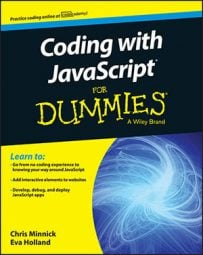##### Coding with JavaScript For DummiesYou can call functions from outside of the function or from within other functions with JavaScript. You can even call a function from within itself. When a function calls itself, it’s using a programming technique called recursion.

You can use recursion in many of the same cases where you would use a loop, except that it repeats the statements within a function.

Here is a simple recursive function. This recursive function has one big problem, however. Can you spot it?

```function squareItUp(startingNumber) {
var square = startingNumber * startingNumber;
console.log(square);
squareItUp(square);
}```

Do you see the issue with this function? It never ends. It will just keep on multiplying numbers together until you stop it.

Running this function will probably crash your browser, if not your computer. No permanent damage will be done, of course, but it’s enough for you to just read the code and notice the problem here.

This example improves upon the squareItUp() function by providing what’s called a base case. A base case is the condition under which a recursive function’s job is done and it should halt. Every recursive function must have a base case.

```function squareItUp(startingNumber) {
square = startingNumber * startingNumber;
if (square > 1000000) {
console.log(square);
} else {
squareItUp(square);
}
}```

There. That’s better! But, this function still has a big problem. What if someone passes a negative number, zero or 1 into it? The result of any of these cases would still be an infinite loop. To protect against such a situation, you need a termination condition. In the code below, a check to make sure that the argument isn’t less than or equal to 1 and that it isn’t something other than a number has been added. In both cases, the function will stop immediately.

```function squareItUp(startingNumber) {
// Termination conditions, invalid input
if ((typeof startingNumber != ‘number’) || (startingNumber <= 1)) {
return - 1; // exit the function
}
square = staringNumber * startingNumber;
//Base condition
if (square > 1000000) {
console.log(square); // Print the final value
} else { // If the base condition isn’t met, do it again.
squareItUp(square);
}
}```